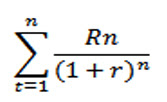### Internal Rate of Return (IRR)

Internal rate of return (IRR) is a discounted cash flow techniques. The discount rate is not specified. The trial and error method is used to find the discount rate which equates the present value of  cash outflows and cash inflows to zero. IRR is the rate where net present value is zero. If the internal rate of return is greater than the required rate of return, the project is acceptable. The cost of capital generally servers as the indicator of the required rate of return.
• Formula, annuity tables and computer programmes are available to calculate internal rate of return. Computer can do seconds where people take hours to calculate IRR.
• The formula for calculating Internal Rate of Return is:where R= Cash Flow, r = discount rate, n = number of years